Origin Of Quantum Mechanics NAT Level – 2

# Origin Of Quantum Mechanics NAT Level – 2

Test Description

## 10 Questions MCQ Test Topic wise Tests for IIT JAM Physics | Origin Of Quantum Mechanics NAT Level – 2

Origin Of Quantum Mechanics NAT Level – 2 for IIT JAM 2023 is part of Topic wise Tests for IIT JAM Physics preparation. The Origin Of Quantum Mechanics NAT Level – 2 questions and answers have been prepared according to the IIT JAM exam syllabus.The Origin Of Quantum Mechanics NAT Level – 2 MCQs are made for IIT JAM 2023 Exam. Find important definitions, questions, notes, meanings, examples, exercises, MCQs and online tests for Origin Of Quantum Mechanics NAT Level – 2 below.
Solutions of Origin Of Quantum Mechanics NAT Level – 2 questions in English are available as part of our Topic wise Tests for IIT JAM Physics for IIT JAM & Origin Of Quantum Mechanics NAT Level – 2 solutions in Hindi for Topic wise Tests for IIT JAM Physics course. Download more important topics, notes, lectures and mock test series for IIT JAM Exam by signing up for free. Attempt Origin Of Quantum Mechanics NAT Level – 2 | 10 questions in 45 minutes | Mock test for IIT JAM preparation | Free important questions MCQ to study Topic wise Tests for IIT JAM Physics for IIT JAM Exam | Download free PDF with solutions
 1 Crore+ students have signed up on EduRev. Have you?
*Answer can only contain numeric values
Origin Of Quantum Mechanics NAT Level – 2 - Question 1

### The life time of a nucleus in excited state is 10–12s. The uncertainty in the energy and frequency of γ-ray photon emitted by it. (h = 6.63 × 10–34 Js) (in units of 1011 Hz) is a × 1011Hz. Find a.

Detailed Solution for Origin Of Quantum Mechanics NAT Level – 2 - Question 1

From the energy time uncertainty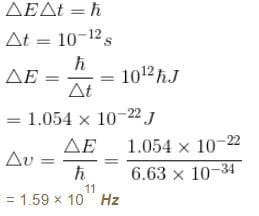The correct answer is: 1.59 x 10^11 Hz

*Answer can only contain numeric values
Origin Of Quantum Mechanics NAT Level – 2 - Question 2

### The number of photon emitted per second by a 40W source of mono chromatic light of wavelength 6000Å is given by x × 1020. Find the value of x.

Detailed Solution for Origin Of Quantum Mechanics NAT Level – 2 - Question 2

*Answer can only contain numeric values
Origin Of Quantum Mechanics NAT Level – 2 - Question 3

### For what wavelength of photon does compton scattering result in a photon whose energy is one half that of the original photon at a scattering angle of 45º? Give the answer as [a × 10–3 Å] . Find the value of a.

Detailed Solution for Origin Of Quantum Mechanics NAT Level – 2 - Question 3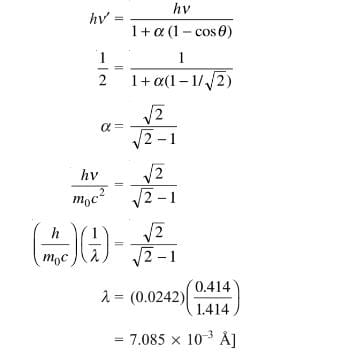The correct answer is: 7.085

*Answer can only contain numeric values
Origin Of Quantum Mechanics NAT Level – 2 - Question 4

For what value of an electron’s speed will its de-Broglie wavelength be same as the compton wavelength? (in terms of c)

Detailed Solution for Origin Of Quantum Mechanics NAT Level – 2 - Question 4

de Broglie wavelength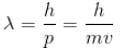Compton wavelength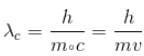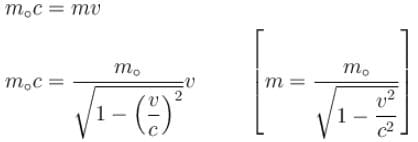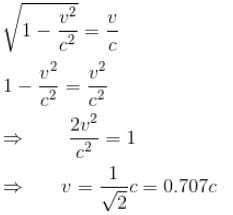*Answer can only contain numeric values
Origin Of Quantum Mechanics NAT Level – 2 - Question 5

Gamma ray photons of energy 1.02 MeV are scattered from electrons which are initially at rest. Find the angle for symmetric scattering at this energy (in degrees)

Detailed Solution for Origin Of Quantum Mechanics NAT Level – 2 - Question 5

For symmetric scattering θ = φ
From the relation between  θ & φ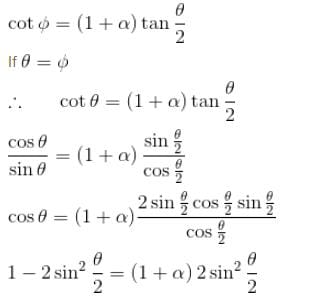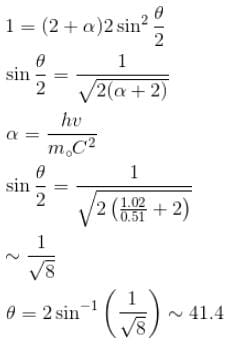*Answer can only contain numeric values
Origin Of Quantum Mechanics NAT Level – 2 - Question 6

The average life time of an excited atomic state is 10–8s. If the wavelength of the spectral line associated with the transition from this state to the ground state is 6000 Å. What will be the width of this line? (in femtometer)

Detailed Solution for Origin Of Quantum Mechanics NAT Level – 2 - Question 6

Average life time = Δt = uncertainty in time = 10–8s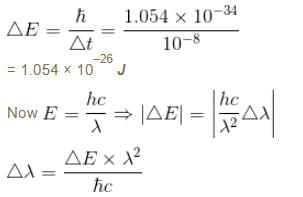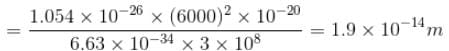width of the line = 1.9 × 10–14 m

*Answer can only contain numeric values
Origin Of Quantum Mechanics NAT Level – 2 - Question 7

If the X-ray photon is scattered at angle of 180° and electron recoils with an energy of 4 keV. Then calculate the wavelength of the incident photon (in Å) in angstroms.

Detailed Solution for Origin Of Quantum Mechanics NAT Level – 2 - Question 7

K.E. of recoiled e is
E = 4 × 103 × 1.6 × 10–19 Joules = 6.4 × 10–16 J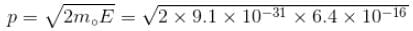= 34.13 × 10–24 kg m/s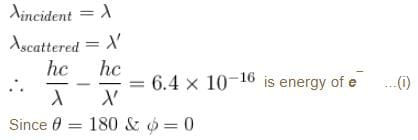From conservation of momentum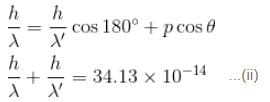solving (i) & (ii)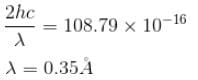*Answer can only contain numeric values
Origin Of Quantum Mechanics NAT Level – 2 - Question 8

The minimum kinetic energy of an alpha particle that can exist in a nucleus (use uncertainty principle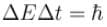)  (Radius of nucleus = 10–14m) (in keV)

Detailed Solution for Origin Of Quantum Mechanics NAT Level – 2 - Question 8

Radius of nucleus = 10–14 m
∴ Uncertainty in position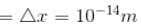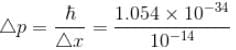= 1.054 × 10–20 kg m/s
Mass of α particle = 4mp = 4 × 1.67 × 10–27 kg
0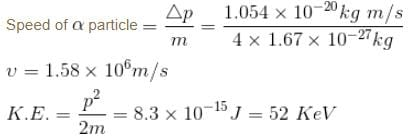*Answer can only contain numeric values
Origin Of Quantum Mechanics NAT Level – 2 - Question 9

The intensity of scattered monochromatic beam of X-rays is plotted as a function of wavelength. There are 2 peaks observed. The distance between the 2 peaks is 0.024 Å. Find the angle (in degrees) at which the X-rays are scattered.

Detailed Solution for Origin Of Quantum Mechanics NAT Level – 2 - Question 9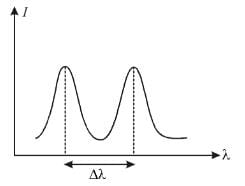Distance between two peaks = Δλ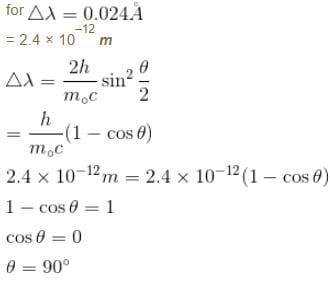*Answer can only contain numeric values
Origin Of Quantum Mechanics NAT Level – 2 - Question 10

Calculate the ground state energy of a Helium atom, using the uncertainty principle. (in eV)

Detailed Solution for Origin Of Quantum Mechanics NAT Level – 2 - Question 10

For a helium atom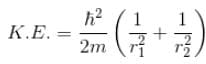Because momentum of electron 1,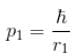and momentum of electron 2,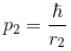p1,  p2 is the spread in momentum corresponding to electron 1 & 2 respectively.

r1r2  is the localization of electron 1 & 2 respectively.

Total energy, E = K.E + Interaction energy of e1 and e2 + Interaction energy between nucleus and electrons

Interaction Energy between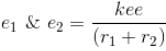Since the separation between r1 and r2 is of the order r1 + r2 and k = 1 in natural system of units.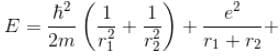Interaction between nucleus and electron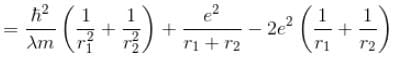For the ground state energy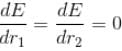since in the ground state, Energy is minimum.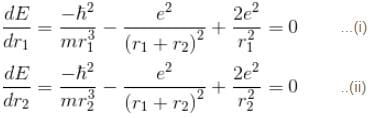solving these two equations, we get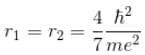substituting r1 and r2 in the expression for E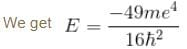Emin = –10.34 eV
Put  m = 9.1 × 10–31 kg
e = 1.6 × 10–19C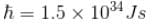## Topic wise Tests for IIT JAM Physics

217 tests
 Use Code STAYHOME200 and get INR 200 additional OFF Use Coupon Code
Information about Origin Of Quantum Mechanics NAT Level – 2 Page
In this test you can find the Exam questions for Origin Of Quantum Mechanics NAT Level – 2 solved & explained in the simplest way possible. Besides giving Questions and answers for Origin Of Quantum Mechanics NAT Level – 2, EduRev gives you an ample number of Online tests for practice

217 tests